```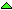;; constants
;; Fixed second counts and calendar facts.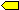(define seconds-in-a-normal-year 31536000)(define seconds-in-a-leap-year 31622400)(define seconds-in-a-week 604800)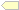(define seconds-in-a-day 86400)(define seconds-in-an-hour 3600)(define base-year 1970)(define month-length-normal-year
(vector 31 28 31 30 31 30 31 31 30 31 30 31));; functions
;; The section of functions.
; Return whether y is a leap year(define (leap-year y)
(cond ((= (modulo y 400) 0) #t)
((= (modulo y 100) 0) #f)
((= (modulo y 4) 0) #t)
(else #f)))

; Return a list of year and remainding seconds of the second
; counter n.(define (years-and-seconds n)
(cycle-years 0 base-year n))

; Count years by increasing n and decreasing u until u is less than the seconds in a year.
; Both n and u are integeres representing a number of seconds.
; Returns a list of year and remaining seconds (less than a year).(define (cycle-years n year u)
(let ((year-length (if (leap-year year)
seconds-in-a-leap-year
seconds-in-a-normal-year)))
(if (< u year-length)
(list year u)
(cycle-years (+ n year-length) (+ 1 year) (- u year-length)))))

; Return the number days, hours, minutes and seconds in second count n.
; n is less than the number of seconds in a year(define (how-many-days-hours-minutes-seconds n)
(let* ((days (quotient n seconds-in-a-day))    ;(n-rest-1 (modulo n seconds-in-a-day))  ;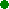(hours (quotient n-rest-1 seconds-in-an-hour))  ;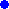(n-rest-2 (modulo n-rest-1 seconds-in-an-hour)) ;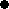(minutes (quotient n-rest-2 60)) ;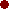(seconds (modulo n-rest-2 60)))  ;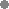(list days hours minutes seconds)))

; Return a list of day and moth given a number of days in year.
; Day-count is a number of days in a year.(define (day-and-month day-count year)
(day-and-month-help 0 1 year (+ 1 day-count)) )(define (day-and-month-help n m y c)
(if (<= c (days-in-month m y))
(list c m)
(day-and-month-help (+ n (days-in-month m y)) (+ m 1)
y (- c (days-in-month m y)))))

; Return the number of days in month and year(define (days-in-month month year)
(if (= month 2)
(if (leap-year year) 29 28)
(vector-ref month-length-normal-year (- month 1))))

; Return a list of year month day hours minutes seconds from n,
; which represents the number of seconds elapsed since January 1, 1970.(define (time-decode n)
(let* ((year-seconds
(years-and-seconds n)) ;(year (car year-seconds))
(days-hours-minutes-seconds   ;(hours (second days-hours-minutes-seconds))
(minutes (third days-hours-minutes-seconds))
(seconds (fourth days-hours-minutes-seconds))
(day-month
(day-and-month (first days-hours-minutes-seconds) year)) ;(day (first day-month))
(month (second day-month)))
(list year month day hours minutes seconds)))

```